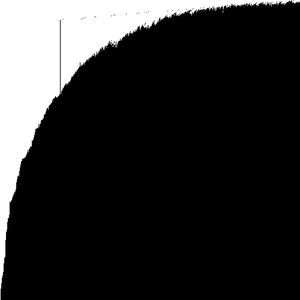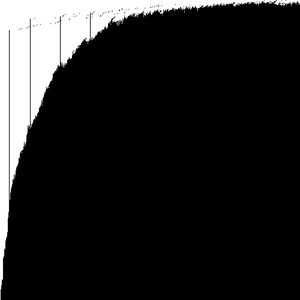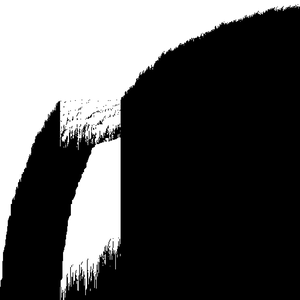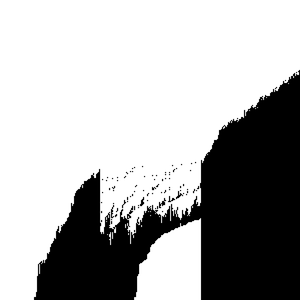# Inhomogeneous space PushTASEP and its new multilayer version

### Definition of the model

Fix a positive function $\xi(x)$, $x\in\mathbb{Z}_{\ge0}$, separated from $0$ and $\infty$. Fix a number of layers $k$, and consider the following $k$-layer particle configuration $x^{(j)}_1<x^{(j)}_2<x^{(j)}_3<\ldots$, $j=1,\ldots,k$. This particle configuration evolves in continuous time. The initial condition is the densely packed (step) one,

$$x_i^{(j)}=i,\qquad i=1,2,\ldots,\qquad j=1,\ldots,k.$$

The evolution is as follows. At each site $y\in\mathbb{Z}_{\ge0}$ there is an independent exponential clock with rate $\xi(y)$ (so, mean waiting time $1/\xi(y)$). This rate does not depend on the layer’s number. When the clock at site $y$ rings:

• if there is a particle at site $y$ at layer $j=1,\ldots,k$, choose the minimal such $j$. The particle $x_i^{(j)}=y$ (with suitable $i$) jumps to the right by one. If the destination is occupied, the immediate right neighbor $x_{i+1}^{(j)}$ also jumps to the right by one, and so on, until an empty spot. If there is no empty spot, then we simply shift the right-infinite densely packed cluster of particles to the right by one.
• if there is no particle at site $y$ at layer $j=1,\ldots,k$, then nothing happens (though by increasing $k$, we can always find the layer at which the dynamics occurs).

The dynamics on the first layer $\{x_i^{(1)}\}$ is simply the PushTASEP (= long-range TASEP) studied, e.g., in . The space-inhomogeneous version of the PushTASEP is studied in .

### Sampling algorithm

To sample the system, we choose a fixed size $n$ (so we consider the behavior on $\{1,2,\ldots,n \}\subset\mathbb{Z}_{\ge0}$), the number of layers $k$, and the inhomogeneity function $\xi(\cdot)$.

Since the dynamics is a continuous time Markov process, we sample it directly, using exponential clocks. In more detail, we sample an independent exponential random variable with mean $1/\xi(y)$ for each $y=1,\ldots,n$. Then we choose the minimal of these waiting times, and

• decrease all other waiting times by this minimal amount,
• perform the jumping/pushing at this site on a suitable layer,
• increment the global time,
• resample the waiting time that was used.

### Data file format

The data files are Mathematica readable 2d integer arrays of the form

{{a,b,c,d},{e,f,g,h},{x,y,z,t}}


where $k$ is the number of blocks ($3$ in the above example), and $n$ is the length of a block ($4$ in the above example). Each element of the array is an integer $\in \{0,1 \}$, where $1$ means that there is a particle at the corresponding site and the corresponding layer, and $0$ means the absence of such particle. In other words, the data reads the configuration layer by layer, starting from the first layer, and shows occupation variables.

There are no spaces or line breaks in the file.

The plots show the first layer on top, layers correspond to horizontals. Black squares mean particles, and white squares mean empty space.

## code • (Main GitHub repo)

(note: parameters in the code might differ from the ones in simulation results below)

## simulation results

1. ##### Homogeneous case, size 100 • (data: 20 KB) • (graphics: 5.7 KB)
$n=100$, $k=100$, $t=100$, $\xi(y)\equiv 1$2. ##### Homogeneous case, size 500 • (data: 489 KB) • (graphics: 86 KB)
$n=500$, $k=500$, $t=500$, $\xi(y)\equiv 1$3. ##### A larger homogeneous picture showing that there is no densely packed region on the first layer • (data: 117 KB) • (graphics: 21 KB)
$n=400$, $k=150$, $t=100$, $\xi(y)=1$4. ##### Slow bond at 100 • (data: 489 KB) • (graphics: 86 KB)
$n=500$, $k=500$, $t=500$, $\xi(y)=\mathbf{1}_{y\ne 100}+\frac{\mathbf{1}_{y=100}}{10}$5. ##### Cluster of 5 slow bonds around 100 • (data: 489 KB) • (graphics: 86 KB)
$n=500$, $k=500$, $t=500$, $\xi(y)=\mathbf{1}_{y\notin [96,100]}+\frac{\mathbf{1}_{y\in [96,100]}}{10}$6. ##### Slow bonds at 15, 50, 100, and 150 • (data: 489 KB) • (graphics: 86 KB)
$n=500$, $k=500$, $t=500$, $\xi(y)=1-\frac{9}{10}(\mathbf{1}_{y=15}+\mathbf{1}_{y=50}+\mathbf{1}_{y=100}+\mathbf{1}_{y=150})$7. ##### Slow zone from 100 to 200 • (data: 489 KB) • (graphics: 86 KB)
$n=500$, $k=500$, $t=500$, $\xi(y)=\mathbf{1}_{y\notin[100,200]}+\frac{\mathbf{1}_{100\le y\le 200}}{10}$8. ##### Fast zone from 100 to 200 • (data: 489 KB) • (graphics: 86 KB)
$n=500$, $k=500$, $t=500$, $\xi(y)=\mathbf{1}_{y\notin[100,200]}+5\cdot \mathbf{1}_{100\le y\le 200}$9. ##### Much faster zone from 100 to 200 • (data: 489 KB) • (graphics: 90 KB)
$n=500$, $k=500$, $t=500$, $\xi(y)=\mathbf{1}_{y\notin[100,200]}+25\cdot \mathbf{1}_{100\le y\le 200}$10. ##### Much faster zone from 100 to 200, another simulation • (data: 176 KB) • (graphics: 36 KB)
$n=300$, $k=300$, $t=500$, $\xi(y)=\mathbf{1}_{y\notin[100,200]}+25\cdot \mathbf{1}_{100\le y\le 200}$11. ##### A mix of fast and slow zones • (data: 396 KB) • (graphics: 74 KB)
$n=450$, $k=450$, $t=500$, $\xi(y)=\frac{\mathbf{1}_{y\in[50,150]}}5+25\cdot\mathbf{1}_{y\in[300,400]} +\mathbf{1}_{y\notin[50,150]\cup[300,400]}$12. ##### Slow zone followed by a fast • (data: 118 KB) • (graphics: 27 KB)
$n=200$, $k=300$, $t=150$, $\xi(y)=\mathbf{1}_{y\le 150}+20\cdot \mathbf{1}_{y> 150}$13. ##### Fast bond at 50 • (data: 118 KB) • (graphics: 25 KB)
$n=200$, $k=300$, $t=150$, $\xi(y)=\mathbf{1}_{y\le 150}+20\cdot \mathbf{1}_{y> 150}$14. ##### Periodic rates • (data: 235 KB) • (graphics: 47 KB)
$n=300$, $k=400$, $t=300$, $\xi(y)=\mathbf{1}_{y\text{ odd}}+5\cdot \mathbf{1}_{y\text{ even}}$## references

1. A. Borodin, P. Ferrari, Large time asymptotics of growth models on space-like paths I: PushASEP, Electron. J. Probab. (2008), vol. 13, 1380-1418 • https://arxiv.org/abs/0707.2813http://emis.ams.org/journals/EJP-ECP/article/download/541/541-1801-1-PB.pdf
2. L. Petrov, In preparation (2018)